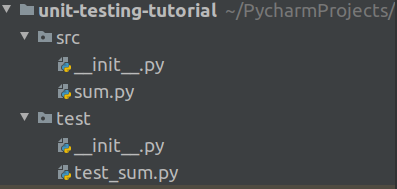# Simple All You Need Unit Testing### Intro

I discovered something interesting today while unit testing my Python code.

It seems that a boolean, call it var1 variable passes the `isinstance(*var1*, int)` check.

I was summing some numbers and at some point realized that the code could sum numbers (int variables) and boolean variables. This was problematic for me and it should not have happened. It appears that Javascript does exactly the same, while Ruby fails with an error.

### Technically Speaking

`isinstance(*var1*, int)` returns `True` for every value of var1 which is an integer. It was counterintuitive for me to see it return `True` when the value of var1 is NOT an integer but a boolean. Python’s syntax is very intuitive and lacks the complexity of programming languages such as Java. This is why I love Python for. There is no need for declaring the type of the variable - as the type of the variable is based on its current value.

Interestingly, when working with datasets loaded into matrices in pandas, I count how many missing values there are by simply summing all the missing values. This should have been a hint as I was getting the count of the missing values in a column of the dataset by summing all the values `True` (True when a cell is empty - e.g. there is a missing value, and Flase - otherwise). In these cases, the sum of many `Trues` would return an integer number to me. Uh oh…

This got me thinking that:

A good way to avoid such misunderstandings is to have solid coverage of your code by unit tests.

This is something I apparently did not have since my code added a boolean to an integer. Let me explain briefly how unit testing works and how to strive to make is more complete over time.

### Unit Testing

This is a quick and more detailed introduction to unit testing than what tutorials you see on the Internet (one of which is in the Further Reading section below).

In Python, I use the commonly-known library `unittest`.

1. Setup

This is how my setup and file structure look like:I have all of my code in the `src` folder, and all of my unit tests in the `test` folder. A file `__init__.py` exists in both folders as I would like Python to see them as modules and import functions from the .py file contained within these modules.

2. The code to be tested

I create a function which I would like to call in my test code which sums two integers.

``````def sum_two_integers(i, j):
if isinstance(i, int) and isinstance(j, int):
return sum([i, j])
else:
return None
``````

I store it in the `sum.py` file.

Note here that I am already making sure that the two variables are integers (or so I thought).

3. The tests

This is how `test_sum.py` looks like:

``````import unittest
import sys
sys.path.append('../')
from src.sum import sum_two_integers

class TestSum(unittest.TestCase):

def test_sum(self):
self.assertEqual(sum_two_integers(3, 3), 6, "Should be 6")

def test_sum_2(self):
self.assertEqual(sum_two_integers(1,5), 6, "Should be 6")

if __name__ == '__main__':
unittest.main()

``````

When I run this I get:

``````..
----------------------------------------------------------------------
Ran 2 tests in 0.000s

OK

``````

Great - the two tests (`test_sum` and `test_sum_2`) have completed successfully.

Note: `sys.path.append('../')` is needed so that I can all the module `src` and import the `sum_two_integers` function from there.

``````import sys
sys.path.append('../')
``````

What else?

The current unit test setting is the following that it does not check for any cases when either of the variables to sum are not integers. Let’s add 3 more tests to cover these cases: the first variable is an integer and the second one is not, the second variables in an integer and the first variable is not, and finally - both varialbes are not integers.

``````import unittest
import sys
sys.path.append('../')
from src.sum import sum_two_integers

class MyNewClass:
'''I have created a new class'''
pass

class TestSum(unittest.TestCase):

def test_sum(self):
self.assertEqual(sum_two_integers(3, 3), 6, "Should be 6")

def test_sum_2(self):
self.assertEqual(sum_two_integers(1,5), 6, "Should be 6")

def test_sum_3(self):
self.assertEqual(sum_two_integers(1,'Ok'), None, "Should be None") # passed a string

def test_sum_4(self):
self.assertEqual(sum_two_integers(True,5), None, "Should be None") # passed a boolean value

def test_sum_5(self):
self.assertEqual(sum_two_integers(MyNewClass(),'I am here.'), None, "Should be None") # passed an object and a string

if __name__ == '__main__':
unittest.main()
``````

I ran the unit tests again using the `python3 test_sum.py` command and this is the result I got:

``````...F.
======================================================================
FAIL: test_sum_4 (__main__.TestSum)
----------------------------------------------------------------------
Traceback (most recent call last):
File "test_sum.py", line 22, in test_sum_4
self.assertEqual(sum_two_integers(True,5), None, "Should be None")
AssertionError: 6 != None : Should be None

----------------------------------------------------------------------
Ran 5 tests in 0.000s

``````

As we can see `test_sum_4` failed as Python returned 6 instead of None. To fix this issue the original `sum_two_integers` function is changed as follows:

``````def sum_two_integers(i, j):
if isinstance(i, int) and isinstance(j, int) and (not isinstance(i, bool)) and (not isinstance(j, bool)):
return sum([i, j])
else:
return None
``````

All unit tests pass after this update:

``````.....
----------------------------------------------------------------------
Ran 5 tests in 0.000s

OK

``````

### Going further

It makes a lot of sense to use unit testing in your big coding projects - which is also test-driven development. It is a great habit to have and can hep you deliver production-ready code to amaze the world.